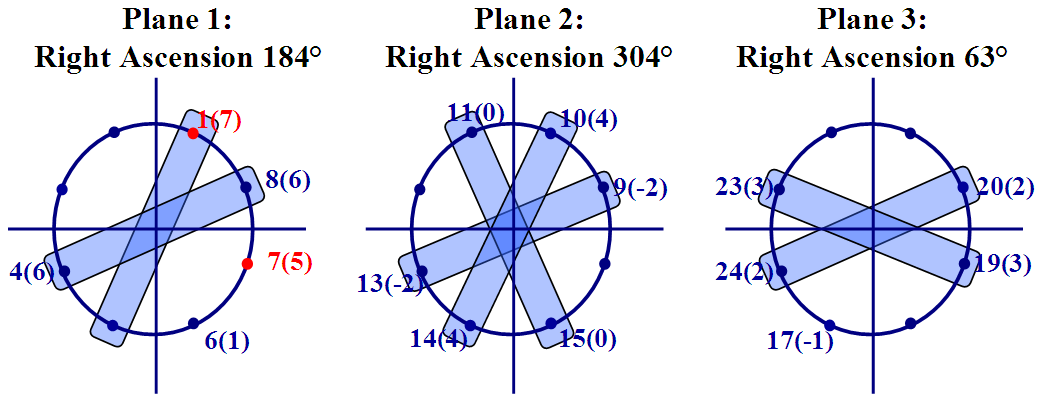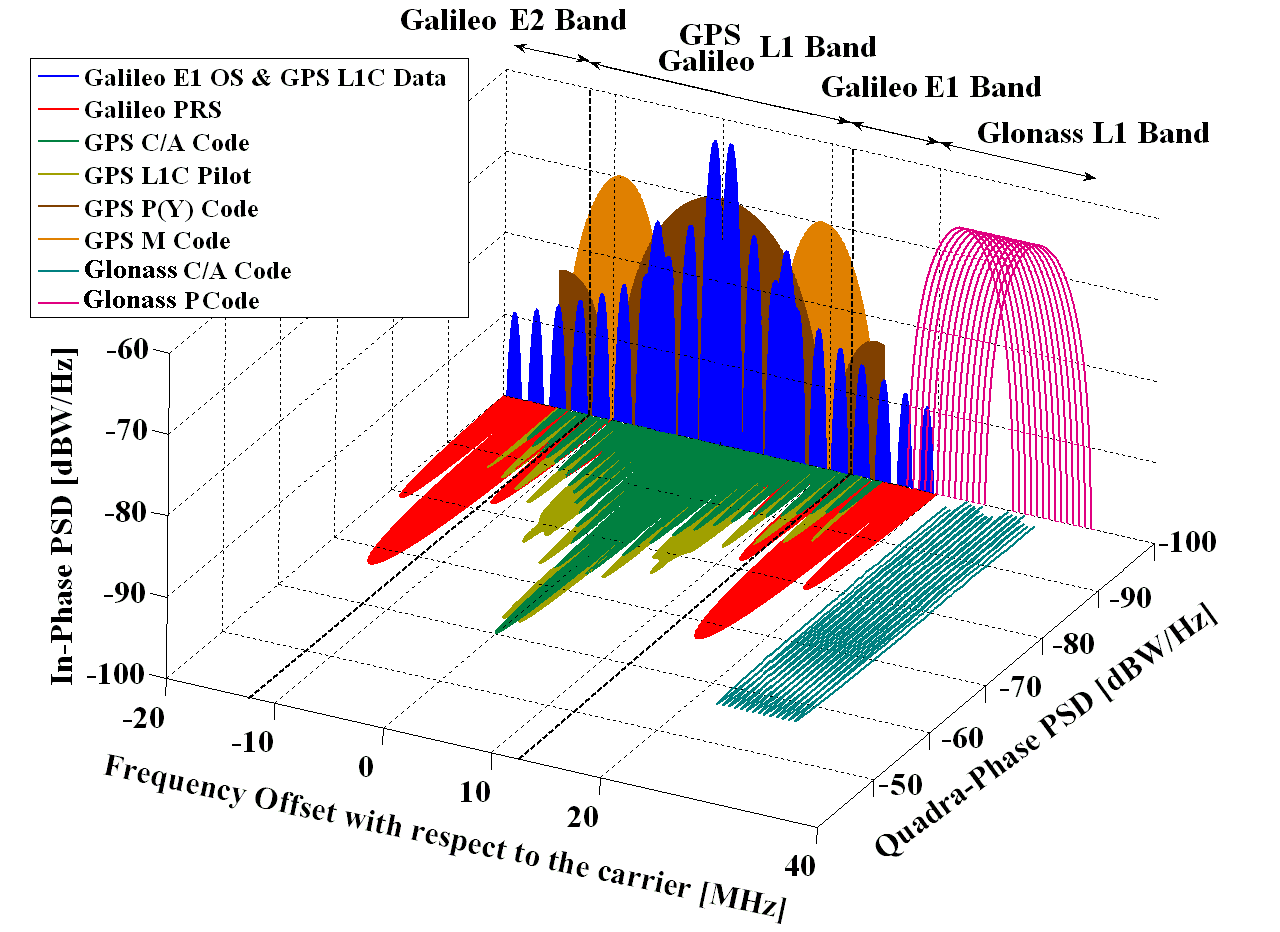If you wish to contribute or participate in the discussions about articles you are invited to contact the Editor

# GLONASS Signal PlanFundamentals
Title GLONASS Signal Plan
Author(s) J.A Ávila Rodríguez, University FAF Munich, Germany.
Year of Publication 2011

Unlike GPS and the other GNSSs, GLONASS uses Frequency Division Multiple Access (FDMA) rather than Code Division Multiple Access (CDMA) for its legacy signals transmission. Originally, the system transmitted the signals within two bands: Ll, 1602.0–1615.5 MHz, and L2, 1246.0–1256.5 MHz, at frequencies spaced by 0.5625 MHz at L1 and by 0.4375 MHz at L2. This arrangement provided 25 channels, so that each satellite in the full 24-satellite constellation could be assigned a unique frequency.

The system now uses only 14 primary frequency channels with k values ranging from –7 to +6, including two channels for testing purposes (currently –5 and –6). (The +7 channel has also been used in the past for testing purposes.) How can 24 satellites get by with only 14 channels? The solution is for antipodal satellites — satellites in the same orbit plane separated by 180 degrees in argument of latitude — to share the same channel. This approach is quite feasible because a user at any location on Earth will never simultaneously receive the signals from such a pair of satellites. The red slots indicate that the satellite is in maintenance. Blue means correct operation.Figure 1: Antipodal Assignment of GLONASS Satellites. The parameter i(k) indicates that the satellite in almanac slot i transmits on frequency number k.

Like the legacy GPS signals, the GLONASS signals include two pseudorandom noise (PRN) ranging codes: ST (for Standartnaya Tochnost or Standard Precision) and VT (for Visokaya Tochnost or High Precision) similar to the GPS C/A- and P-codes, respectively (but at half the chipping rates), modulated onto the L1 and L2 carriers. The GLONASS ST code is 511 chips long with a rate of 511 kilochips per second, giving a repetition interval of 1 millisecond. The VT-code is 33,554,432 chips long with a rate of 5.11 megachips per second. The code sequence is truncated to give a repetition interval of 1 second. Unlike GPS satellites, all GLONASS satellites transmit the same codes.

The nominal values of the FDMA L1, L2 and L3 carrier frequencies are defined as:

$\begin{array}{r} f_{k_L1} = f_{0_{L1}}+ k\Delta f_{L1}\\[0.3cm] f_{k_L2} = f_{0_{L2}}+ k\Delta f_{L2}\\[0.3cm] f_{k_L3} = f_{0_{L3}}+ k\Delta f_{L3}\\ \end{array} \qquad \mbox{(1)}$
where:
$k$ represents the frequency channel,
$f_{0_{L1}}$ = 1602 MHz for the GLONASS L1 band,
$\Delta f_{L1}$ = 562.5 kHz frequency separation between GLONASS carriers in the L1 band,
$f_{0_{L2}}$ = 1246 MHz for the GLONASS L2 band,
$\Delta f_{L2}$ = 437.5 kHz frequency separation between GLONASS carriers in the L2 band,
$f_{0_{L3}}$ = 1201 MHz for the GLONASS L3 band, and
$\Delta f_{L3}$ = 437.5 kHz frequency separation between GLONASS carriers in the L3 band.

The new GLONASS-K satellites transmit additional signals. GLONASS-K1 transmit a CDMA signal on a new L3 frequency (1202.025 MHz), and GLONASS-K2, in addition, will feature CDMA signals on the L1 and L2 frequencies.

Like GPS and the other GNSSs, the GLONASS signals also contain navigation messages providing satellite orbit, clock and other information. Separate 50-bits-per-second navigation messages are modulo-2 added to the ST- and VT-codes. The ST-code message includes satellite clock epoch and rate offsets from GLONASS System Time; the satellite ephemeris given in terms of the satellite position, velocity and acceleration vectors at a reference epoch; and additional information such as synchronization bits, data age, satellite health, offset of GLONASS System Time from Coordinated Universal Time (UTC) as maintained by the National Metrology Institute of the Russian Federation UTC(SU) as part of the State Time and Frequency Service, and almanacs (approximate ephemerides) of all other GLONASS satellites.

To have a clearer insight into how the spectra of the GLONASS signals look like, we study next all the bands in detail.

## GLONASS L1 Band

The transmitted navigation signal is in both services of L1 a bipolar phase-shift key (BPSK) waveform with clock rates of 0.511 and 5.11 MHz for the standard and accuracy signals respectively. The L1 signal is modulated by the Modulo-2 addition of the pseudo random (PR) ranging code, the digital data of the navigation message and an auxiliary meander sequence. All above-mentioned frequencies are generated coherently using a single onboard time/frequency oscillator standard [GLONASS ICD, 2002] . For the case of the standard accuracy signals (C/A), the PR ranging code is a sequence with length the maximum of a shift register (m-sequence) and a period of 1 millisecond with bit rate of 511 kbps. The navigation message is sent at 50 bps and the auxiliary meander sequence at 100 Hz.

Moreover, it is important to note that the GLONASS FDMA L1 band does not exactly coincide with the GPS and Galileo L1 band. In fact, the GLONASS L1 band ranges from 1592.9525 MHz to 1610.485 MHz when only the 14 channels k = -7...+6 are employed. In the next figures, each of the channels was filtered to only transmit the main lobe of the BPSK signal and the PSD was normalized to have unit power within the corresponding transmission bandwidth.

The PSDs of the GLONASS signals are shown in the following figure:

Once again, in order to have a clearer picture of how overcrowded the RNSS bands are becoming as more and more countries claim their rights to have their own GNSS, the following figure shows GPS, Galileo and GLONASS signals in the E1/L1 band.Figure 3: Spectra of GPS, Galileo and GLONASS Signals in E1/L1.

It is important to note that the GPS L1C pilot and data signals are shown in quadrature in the figure although according to [GPS ICD-800, 2006] the final phasing is still open. To finalize some details on the technical characteristics of the GLONASS L1 signals are presented next:

It is important to note that unlike for the case of GPS and Galileo in the previous chapters, the frequencies do not have to be multiplied by the factor 1.023.

CDMA Open Service Navigation Signal in L1 frequency band is called L1OC. It is transmitted on carrier frequency

    fL1 = 1565 fb = 313 x 5.115 MHz = 1600.995 MHz


and consists of two components of the same power: L1OCd (data component) and L1OCp (pilot component). These components are obtained by chip by chip time-division multiplexing (TDM) of two pseudorandom noise sequences (PRNs). L1OC signal is in phase quadrature with L1SC signal, which is delayed by 90º.

### L1OCd

Modulation sequence of symbols of L1OCd signal is the module-2 sum of PRNL1OCd chip stream clocked at fT1/2 = 0.5115 MHz, overlay code (OC1) symbol stream clocked at 500 sps, and convolution encoder (CE) symbol stream clocked at 250 sps .

Navigation message of L1OCd signal is transmitted as a continuous sequence of strings of non-fixed and non-predetermined structure.

Navigation message of L1OCd signal is transmitted at 125 bps. It consists of 250-bit strings of 2 second duration, as well as of 125 and 274 bit anomalous strings of 1 and 3 second duration, respectively.

### L1OCp

Modulation sequence of symbols of L1OCd signal is the module-2 sum of PRNL1OCp chip stream clocked at fT1/2 = 0.5115 MHz, and meander sequence (MS) clocked at: 2 fT1 = 2.046 MHz.

The MS is a 0101 periodic sequence, synchronized with PRNL1OCp chips and transmitted with most significant bits ahead. MS is intended to form BOC (1, 1) spectrum for L1OCp component.

## GLONASS L2 Band

The transmitted navigation signal is, as also in L1, a bipolar phase-shift key (BPSK) waveform with similar clock rates as in the L1 band. The L2 signal is modulated by the Modulo-2 addition of the PR ranging code and the auxiliary meander sequence. For the case of the standard accuracy signals (C/A), the PR ranging code is a sequence of the maximum length of a shift register (M-sequence) with a period of 1 millisecond and a bit rate of 511 kbps. The navigation message is sent at 50 bps and the auxiliary meander at 100 Hz.

We show in the figure next all the signals of GPS and GLONASS in the L2 band together

To finalize some details on the technical characteristics of the GLONASS L2 signals are presented in the next table:

It is important to note again that unlike for the case of GPS and Galileo, the frequencies do not have to be multiplied by the factor 1.023.

CDMA Open Service Navigation Signal in L2 frequency band is called L2OCp. It is transmitted on carrier frequency (nominal value)

  fL2 = 1220 fb = 244 x 5.115 MHz = 1248.06 MHz


and is multiplexed with L2 CSI signal chip by chip time-division multiplexing of their pseudorandom noise sequences. L2q signal is in phase quadrature with L2SC signal, which is delayed by 90º .

### L2OCp

Modulation sequence of symbols of L2OCp signal is the modulo-2 sum of PRNL2OCp chip stream clocked at fT1/2 = 0,5115 MHz, overlay code OC2 symbol stream clocked at 50 sps, and meander sequence (MS) clocked at 2 fT1 = 2.046 MHz. MS is intended to form BOC (1, 1) spectrum for L2OCp component .

## GLONASS L3 Band

CDMA Open Service Navigation Signal in L3 frequency band is called L3OC. It is transmitted on carrier frequency (nominal value)

 fL3 = 1175 fb = 235 x 5.115 MHz = 1202.025MHz


and consists of two BPSK(10= components of the same power: L3OCd (data component) and L3OCp (pilot component). These components are in phase quadrature with each other and L3OCd is delayed 90º .

### L3OCd

Modulation sequence of symbols of L3OCd signal is the module-2 sum of the PRNL3OCd chip stream clocked at fT1 = 10.23MHz, Barker code (BC) symbol stream clocked at 1000 sps, and convolution encoder (CE) symbol stream clocked at 200 sps. Sequence SL3OCd is used to phase-shift key I-component of carrier frequency in L3 by 180º .

Navigation message of L3OCd signal is transmitted as a continuous sequence of strings of non-fixed and non-predetermined structure.

Navigation message of L3OCd signal is transmitted at 100 bps. Navigation message consists of 300-bit strings of 3-second duration, as well as of 200 and 400-bit anomalous strings of 2 and 4 second duration respectively .

### L3OCp

Modulation sequence of symbols of L3OCp signal is the modulo-2 sum of PRNL3OCp chip stream clocked at fT1 = 10.23MHz and Newman-Huffman code (NH) symbol stream clocked at 1000 sps. Sequence SL3OCp is used to phase-shift key Q-component of carrier frequency in L3 by 180º .